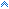Temporal duration discrimination
As in the traditional discrimination task, the subject is presented with two intervals, a standard interval S of duration ts, followed by a variable comparison interval C of duration tc. The duration ts of S is again kept constant across a block of trials, whereas tc varies randomly from trial to trial. The comparison interval is shorter than, equal to, or longer than ts. In contrast to the classical task, however, the subject is required to judge whether S was shorter than, longer than, or equal to C. These three response alternatives will be denoted by “C > S,” “S > C,” and “S 5 C,” respectively. Thus, the ternary- response task allows the estimation of probabilities Pr{“S > C” | ts , t c},
The present experiment employed two standard intervals of 50 and 1,000 msec. For each standard interval there were nine comparison intervals, and subjects were asked to indicate in each trial whether the standard appeared to be shorter than, equal to, or longer than the comparison.Top